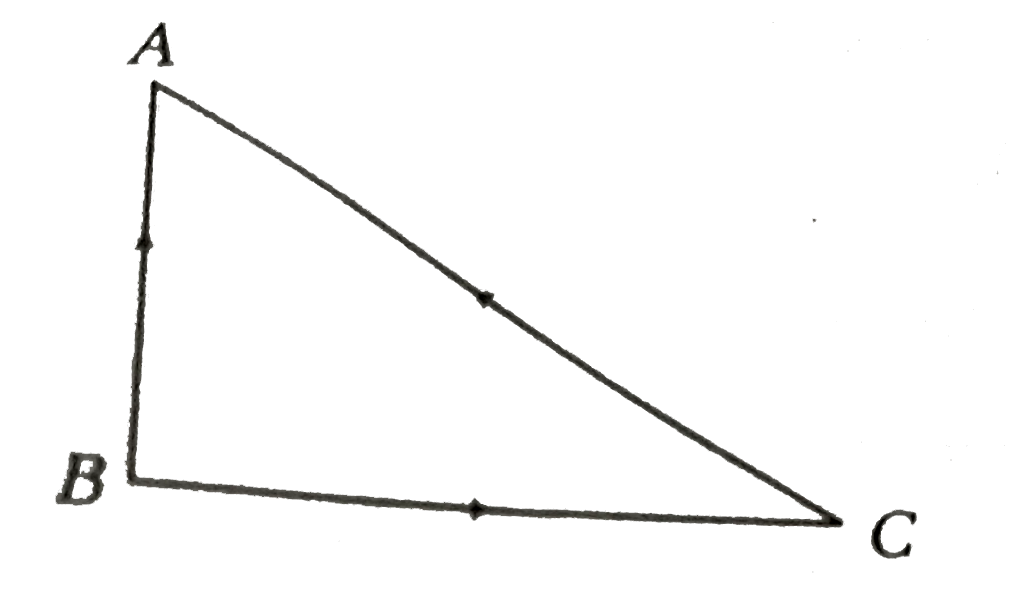# Three rods of identical area of cross-section and made from the same metal from the sides of an isosceles triangle. ABC, right angled at B. The points

52 views
in Physics
closed
Three rods of identical area of cross-section and made from the same metal from the sides of an isosceles triangle. ABC, right angled at B. The points A and B are maintained at temperatures T and sqrt 2T RESPECTIVELY. In the steady state the temperature of the point C is T_(C).
Assuming that only heat conduction takes place , T_(C) / T is equal to
A. 1 / (sqrt2+1)
B. 3 / (sqrt2 + 1)
C. 1 / (2(2sqrt2 - 1)
D. 1 / (sqrt3(sqrt2 -1)

by (90.7k points)
selected

therefore (T_(B) gt T_(A) rArr Heat will flow B to A via two paths (i) B to A
(ii) along BCA as shown.Rate of flow of heat in path BCA will be same
i.e., (Q/t)_(BC) = (Q/t)_(CA)
rArr (K(sqrt(2T) -T_(C))A/(a) = (K(T_(C)-T)A)/(sqrt(2a) rArr (T_(c))/T = (3)/(1+sqrt(2))